# SSC Reasoning Ability Practice Questions (Day-22)

Dear Aspirants, The SSC Exams are going on at national level. Millions of candidates have eagerly prepared for the ongoing SSC exams such as CGL, CHSL, MTS, CPO, JE etc. SSC exams are usually conducted by the Staff Selection Commission to recruit the eligible candidates for various Central government departments. Mostly the selection process for all the SSC exams consists of the single or double phase of written examination based on the examination. Due to that, the written exam consisted of a certain syllabus and exam pattern. By following those regulations, the written exam has been conducted through an online mode. Based on the latest updated exam pattern and syllabus, here we have drafted the Reasoning Ability questions on a daily basis. So aspirants start your practice session with us and mould your skill in a perfect shape.

Start Quiz

1) In the following question, select the number which can be placed at the sign of question mark (?) from the given alternatives.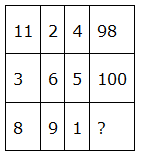(a) 72

(b) 75

(c) 88

(d) 82

2) How many quadrilaterals are there in the given figure?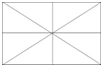(a) 13

(b) 15

(c) 17

(d) 19

3) How many rectangles are there in the given figure?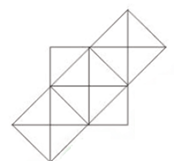(a) 15

(b) 17

(c) 19

(d) 23

4) In each of the following question below are given some statements followed by some conclusions. Taking the given statements to be true even if they seem to be at variance from commonly known facts, read all the conclusions and then decide which of the given conclusion logically follows the given statements.

Statements:

I) All pens are cups.

II) All glasses are cups.

Conclusions:

I) Some pens are glass.

II) Some glasses are cups.

III) Some glasses are pens.

(a) Only conclusion (I) and (III) follow

(b) Only conclusion (I) follows

(c) Only conclusion (II) follows

(d) No conclusion follows

5) In each of the following question below are given some statements followed by some conclusions. Taking the given statements to be true even if they seem to be at variance from commonly known facts, read all the conclusions and then decide which of the given conclusion logically follows the given statements.

Statements:

I) No pens are pencils.

II) No eraser is cup.

III) All pencils are erasers.

Conclusions:

I) Some pens are not erasers.

II) Some erasers are not pens.

III) No pencil is cup.

IV) Some erasers are cups.

(a) Only conclusion (I) and (IV) follow

(b) Only conclusion (I) and (III) follow

(c) Only conclusion (II) and (III) follow

(d) Only conclusion (II) and (IV) follow

6) From the given options, which answer figure can be formed by folding the figure given in the question?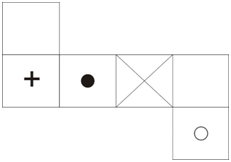(a)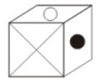(b)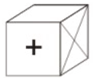(c)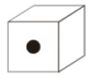(d)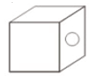7) Two position of a cube are shown below. What will come opposite to face containing ‘&’?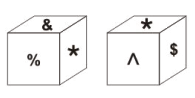(a) ^

(b) %

(c) \$

(d) None of these

8) In the given figure, which number represents plastic tables which are wooden?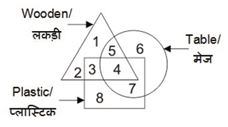(a) 3

(b) 5

(c) 7

(d) 4

9) In the given figure, which number represents brown animals which do not have tail?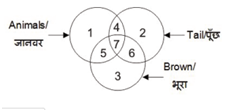(a) 7

(b) 4

(c) 5

(d) 6

10) In the given figure, which number represents the paint brush which is not hair?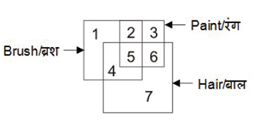(a) 4

(b) 3

(c) 5

(d) 2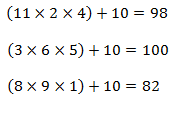15 rectangles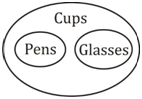I) ×

II)

III) ×

Only conclusion II follows.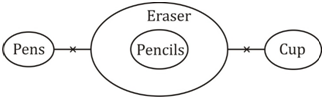I) ×

II)

III)

Only conclusions II and III follow.

Opposite faces are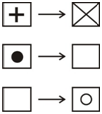Option (a) can be formed by folding

Opposite faces of ‘&’ is ‘^’

4

5